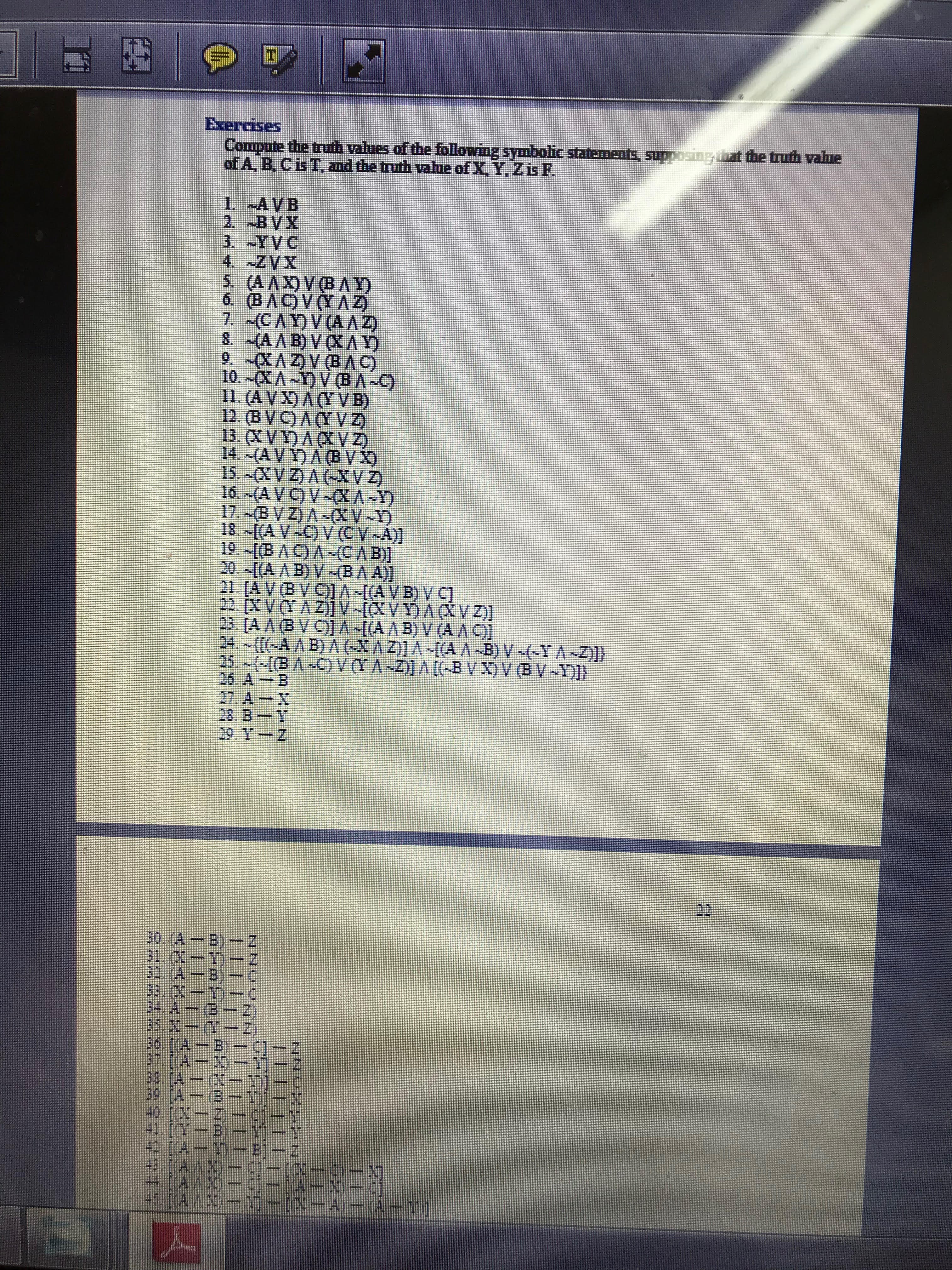# ExercisesCompute the truth values of the followng symbolic statements, supposing atof A B, CisT, and the truth value of X. Y. Zis F.the truth vahue11. (AVD A(TV B)12. (BVC) AYVZ)13 CVD ACV14. (AVDABVI26. AB27, A-28. BT29 Y-Z40 lㅡ피 mcl

Question

#32,36help_outlineImage TranscriptioncloseExercises Compute the truth values of the followng symbolic statements, supposing at of A B, CisT, and the truth value of X. Y. Zis F. the truth vahue 11. (AVD A(TV B) 12. (BVC) AYVZ) 13 CVD ACV 14. (AVDABVI 26. AB 27, A- 28. BT 29 Y-Z 40 l ㅡ피 mcl fullscreen
check_circle

Step 1

Given symbollic statements with B,C and Y,Z shown below. Here the truth value of B,C is Truth and the truth value of Y,Z is False.

Step 2

Here given function --> is implication function and we know Truth implication Truth give us Truth. Using this we have computed A-->B  and we got T.

Step 3

Here given function --> is implication function and we know Truth implication Truth give us Truth. Using this we have computed (A-->B) and by using it and its implication with C we get (A-->...

### Want to see the full answer?

See Solution

#### Want to see this answer and more?

Solutions are written by subject experts who are available 24/7. Questions are typically answered within 1 hour.*

See Solution
*Response times may vary by subject and question.
Tagged in

### Logic